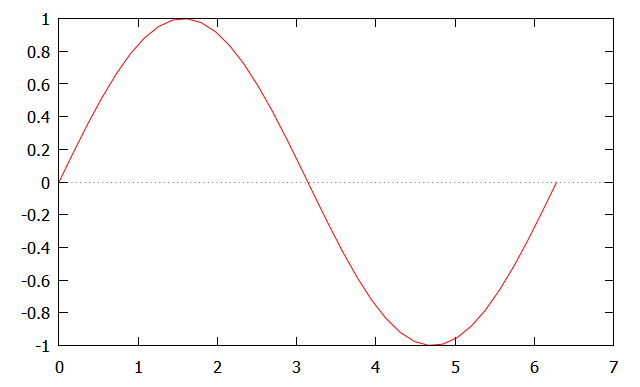Ch Standard Demos
 ISO C90 Standard Wide characters ISO C99 Standard C++ features Complex IEEE floating-point arithmetic Assumed-shape arrays Nested functions Interactive C statement execution Interactive command shell Shell Programming Safe Ch Ch applets String Type Adjustable array bounds Auto array bound checking Socket/Winsock POSIX Unix utilities for Windows Windows GTK+ X/Motif OpenGL Toolkits Demos CGI ODBC ChExcel Ch Professional Demos Computational array Plotting Numerical analysis C LAPACK functions
```Interactive execution of binary commands, and C/C++ expressions, statements, functions,
and programs in a Ch command window are portable across different platforms.

The interactive execution below illustrates how different conversion specifiers
in a format string affect the output.
```
> 2*3+4
10
> int i = 16
> double f = 12.3456
> sizeof(int)
4
> sizeof(i)
4
> sizeof(double)
8
> sizeof(f)
8
> i
16
> 2*i
32
> printf("%d", i)
10
> printf("%5d", i)      // field width 5
10
> printf("%#d", i)
10
> printf("%#x", i)
0xa
> printf("%#X", i)
0XA
> printf("%x", i)
a
> printf("%X", i)
A
> printf("%o", i)
12
> printf("%b", i)
1010
> printf("%f", f)
12.345600
> printf("%lf", f)
12.345600
> printf("%.2lf", f)   // precision with 2 digits after decimal point
12.35
> printf("%8.2lf", f)  // field with of 8
12.35
> printf("%d", f)      // a common mistake using %d for floating-point numbers
2075328197
> printf("%f", i)      // a common mistake using %f for integers
0.000000
>
```

The interactive execution below illustrates a difficult concept
how pointers in C are related to the memory they point to.
```
> int i, *p, **p2     // i is an integer, p pointer, p2 double pointer
> i=10                // i is assigned value 10
10
> p=&i                // p points to address of i
00D847C0
> *p                  // the memory pointed by p has value 10
10
> p2=&p               // p2 points to address of p
00D84D30
> **p2                // the memory pointed by the pointer at p2 has value 10
10
>
```

Commands below illustrate a difficult concept that an array name is a pointer,
whereas a pointer can also be treatd as an array.
```
> int a={1,2,3,4,5}, *p
> p= &a
009BD3D8
> a
3
> *(a+2)
3
> *p
3
> p
3
> p
4
>
```

Commands below illustrate how struct can be handled interactively.
Note that because of alignment, the size of structure tag is 16, not 12.
```
> struct tag {int i; double d;} s
> s.i =20
20
> s.d=30
30.0000
> s
.i = 20
.d = 30.0000
> sizeof(struct tag)
16
>
```
```
```Not only C statements and expressions, but also C functions and  programs,
can be interactively executed in Ch.  All functions in the C standard libraries
can be executed interactively and can be used inside user defined functions.
For example,
```
> srand(time(NULL))
> rand()
4497
> rand()
11439
> double add(double a, double b) {double c; return a+b+sqrt(2);}
> double c
31.4142
```
After the random number generator is seeded with a time value
in srand(time(NULL), the function rand() is called to generate
two random numbers. The function add() which calls the mathematical
function sqrt() is defined at the prompt and then used.

Commands below demonstrates how Ch can be used as a calculator for
scientific numerical computing and visualization.
```
```   ```
> 2*3.4-sin(1.5)                 // calculate 2*3.4-sin(1.5)
7.7975
> array double x
> linspace(x, -3.1416, 3.1416)   // assign array x with values from -3.1416 to 3.1416 linearly
> x                              // display array x
-3.1416 -2.9621 -2.7826 -2.6030 -2.4235 -2.2440 -2.0645 -1.8850 -1.7054 -1.5259
-1.3464 -1.1669 -0.9874 -0.8078 -0.6283 -0.4488 -0.2693 -0.0898 0.0898 0.2693
0.4488 0.6283 0.8078 0.9874 1.1669 1.3464 1.5259 1.7054 1.8850 2.0645 2.2440
2.4235 2.6030 2.7826 2.9621 3.1416
> sin(x)                         // calculate sin(x) for array x
0.0000 -0.1786 -0.3514 -0.5129 -0.6579 -0.7818 -0.8806 -0.9511 -0.9909 -0.9990
-0.9749 -0.9195 -0.8346 -0.7228 -0.5878 -0.4339 -0.2660 -0.0896 0.0896 0.2660
0.4339 0.5878 0.7228 0.8346 0.9195 0.9749 0.9990 0.9909 0.9511 0.8806 0.7818
0.6579 0.5129 0.3514 0.1786 -0.0000
> plotxy(x, sin(x), "Ch plot", "xlabel", "ylabel")
``````
``` ``````The above plot displayed in Windows can be copied and pasted in Word
for documentation and project report.

Commands below illustrate how computational arrays can be handled interactively.
```
> array double a = {1,2,3,4,5,6}, b
> b = a*transpose(a)
14.0000 32.0000
32.0000 77.0000
> b*inverse(b)
1.0000 0.0000
0.0000 1.0000
> a = 100*a + 5
105.0000 205.0000 305.0000
405.0000 505.0000 605.0000
```
In this example, the functions transpose() and inverse() are used to calculate
the transpose and inverse of a matrix, respectively.
```